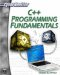# Pointer Operations

Because a pointer is actually an address in memory, rather than a value, it should make sense to you that normal operations on a pointer behave quite differently. For example, with a normal variable if you use the increment operator on it (++), you increase its value by one. With a pointer, you actually move the pointer over a number of bytes equal to the size of the data type. Because an integer is 4 bytes (at least on 32-bit operating systems), if you have an integer pointer and do the increment operation, then it will move the pointer over 4 bytes in memory. So, for example, if you move an int pointer over 4 bytes, it will no longer be pointing at that same int. It will have moved past the 4 bytes occupied by the int variable and will be pointing at some space in memory, the contents of which are not known.

 Watchout! If you move your pointer to some unknown memory location and then do some operation on it, you might be interfering with the operations of some other program on your PC, including the operating system.

To conduct arithmetical operations on pointers is somewhat different than to conduct operations on other data types. To begin with, you can only use addition (+), subtraction (-), increment (++), and decrement () operators. You cannot use multiplication or division on a pointer. Because a pointer contains an address in memory, multiplication and division do not make sense. A pointer holds an address, if you multiply that by 4, you get a number that may represent more memory that your computer even has. But both addition and subtraction have a different behavior with pointers then they do with normal data types. The addition operator causes the pointer to move a certain number of bytes to the right. The subtraction operator causes the pointer to move a certain number of bytes to the left. The number of bytes moved is the number used in the expression times the size of the data type. Consider the following code.

`int a; int *p; p = &a; p =p+2;`

What actually happens in the fourth line is that the pointer moves over bytes. It is not adding 2 to the value of a. It is, instead, pointing to the last 2 bytes of the integer a. This is illustrated in Figure 9.3.Figure 9.3: Adding to a pointer.

You can see that what is occurring is that the pointer is simply moving over. Now this may not seem to be of much use with standard variables, but it is useful with arrays. You will frequently see pointers used in conjunction with arrays. You should recall arrays from Chapter 1. (If you need a refresher, then please return to that chapter and reread the section on arrays.) If you have a pointer with an array, it starts off pointing to the first element of the array. Any arithmetic operations you perform on it will simply move the pointer forward and backward through the array. The following example should help you understand this.

### Example 9.2

Step 1: Enter the following code in your favorite text editor.

`#include <iostream> using namespace std;   int main ()  {   int i;  int *p;  p = i;  for(int j = 0;j<5;j++) {      i[j] = j; p++;      cout << p;      cout << "\n"; } return 0;    }`

Step 3: Run the application. You should see an image much like Figure 9.4.Figure 9.4: Pointers and arrays.

You will notice that what you see is a series of addresses in memory. The pointer points to those addresses, and by moving the pointer you are shifting it to the next element in the array. This is also an excellent time to remind you of the grave danger you can face using a pointer. A pointer will attempt to access whatever memory address you point it to . . . even if that goes beyond the bounds of your array, or even your application! Consider the following example.

### Example 9.3

Step 1: Enter the following code in your favorite text editor.

`#include <iostream> using namespace std;   int main ()  {  int i;  int *p;  p = i;  for(int j = 0;j<5;j++)  {        i[j] = j;  }  for(int k = 0;k<7;k++)  {        p++;        cout << p;        cout << "\n";  } return 0;   }`

Step 2: Compile the code.

This code does not generate an error. C++ allowed you to point the pointer beyond the bounds of the array. So what is the pointer now pointing at? There is no way to know. It might be pointing at another variable in your program, some part of another program running on your PC, or even at some part of the operating system. This is why pointers, if not used carefully, can be quite dangerous.

Remember that programs are not necessarily loaded into contiguous blocks of memory. Right next to your array, in memory, could be a variable from another program, or even from a subroutine of your operating system. Not paying attention to pointers is one way that a C++ programmer can get into a lot of trouble and cause some significant problems on a system. Another thing to remember when working with pointers is to always initialize them to some value. If you simply declare a pointer then try to use it without first pointing to some address in memory, then it will be pointing at an arbitrary address in memory. That could be very problematic. A common technique is to initialize the pointer so that it points at some null value.

` int *x = 0;`C++ Programming Fundamentals (Cyberrookies)
ISBN: 1584502371
EAN: 2147483647
Year: 2005
Pages: 197
Authors: Chuck Easttom